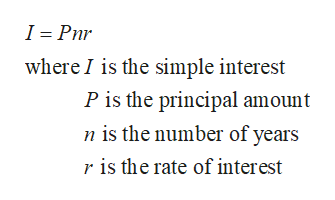# Section 3.1 (17)Compute the specified quantity. Round all answers to the nearest month, the nearest cent, or the nearest 0.001%, as appropriate.You take out a 6-month,  \$5000 loan at 8% annual simple interest. How much would you owe at the end of the 6 months?

Question
1 views

Section 3.1 (17)

Compute the specified quantity. Round all answers to the nearest month, the nearest cent, or the nearest 0.001%, as appropriate.

You take out a 6-month,  \$5000 loan at 8% annual simple interest. How much would you owe at the end of the 6 months?

check_circle

Step 1

Recall the follow...help_outlineImage TranscriptioncloseI = Pnr where I is the simple interest P is the principal amount n is the number of years r is the rate of interest fullscreen

### Want to see the full answer?

See Solution

#### Want to see this answer and more?

Solutions are written by subject experts who are available 24/7. Questions are typically answered within 1 hour.*

See Solution
*Response times may vary by subject and question.
Tagged in

### Math